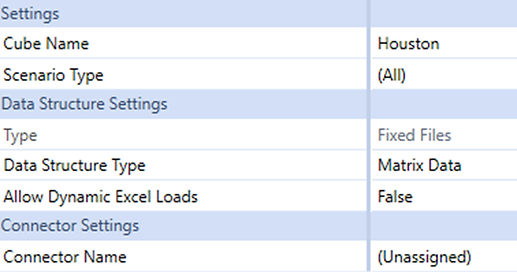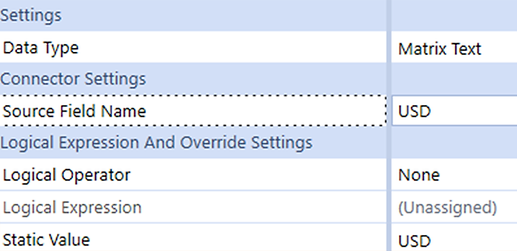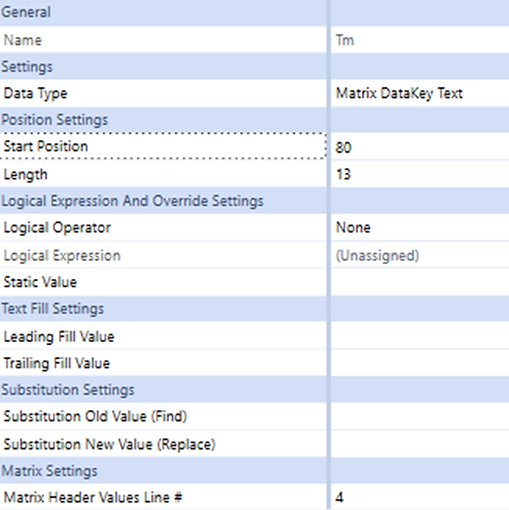top of pagePUBLISHED FEBRUARY 4, 2021

### Multi Columnar Data Load in OneStream

By Zeeshan Ali

For any EPM / CPM project, some of the key discussions revolves around “How do we use Excel files to load data into the application?”’. “Can we make it easier for users to enter data in the system?”.

The data load using Excel may either be a single column of amounts or having amounts in multiple columns. Some examples of having multiple column loads are forecast / budget data for 12 months, data by cost centers / products / departments where cost centers / products etc. are in columns.

For Data Sources in OneStream, Matrix Load enables upload of multiple amount columns for a dimension. Let’s take the budget data for example - the time dimension is in the columns, one column per month.

To use the Matrix Load feature the following settings need to be done in the Data Source:

1.

Set Data Structure Type as “Matrix Data”1.1.    The next step is to identity the data type for every dimension. The Dimension, for which the Matrix Load is to be used, is to be set as “Matrix Type”. For Matrix data load one of these options is to be selected. When the columns are for time / months, use Matrix DataKey Text else use Matrix Text.1.2  Thereafter an additional Matrix setting is to be used for Matrix Load. “The Matrix Header Value Line #” indicates which row to look to for the Dimension being matrixed. For example, if months are listed across columns on line four, and time is the intended Member to be matrixed, a 4 would be entered in this field.2.

Similarly, to load data in different currencies e.g., Functional currency, Transactional, statutory currency matrix load can be used.

In this scenario for each entity, the Trial Balance contains 3 columns for Amount in 3 different currencies - Functional currency, Transactional currency, and Statutory currency. The requirement is to load the amounts in all 3 currencies and analyze the foreign exchange variances.

Below is an example of a trial balance with balances in 3 currencies.In this example, the UD6 Dimension is used for currency. Since there are 3 currencies, UD6 is used thrice and is mapped to the 3 Amount columns. The Data Type is set to “Matrix Text” and the Static Value is used to identify the Currency type. In the below screenshot, static value is “TC” which is mapped to the “Amount in TC” column.After import and transformation, the data will appear as below:3.

Let us have a look at a Matrix Excel Template. For Excel upload OneStream uses property tokens for dimensions to load to Forms.

To load budget data to multiple periods at one go, the Time Dimension Token is combined with the amount to identify which column should loaded to what period. The example below indicates the Amount Column using AMT# and then specifying to which Time Members the Amount detail belongs.Matrix load makes data import convenient and easy to use where multiple amounts are to be loaded for a single dimension, as explained above.

This feature provides flexibility to the users to load excel files as generated from the source systems for further analysis in OneStream.

Converting existing excel files into upload formats gives users the flexibility of excel as well as the benefits of OneStream with minimal changes for users.

- Zeeshanbottom of page